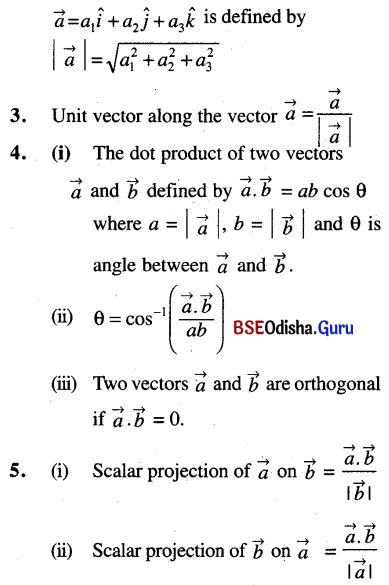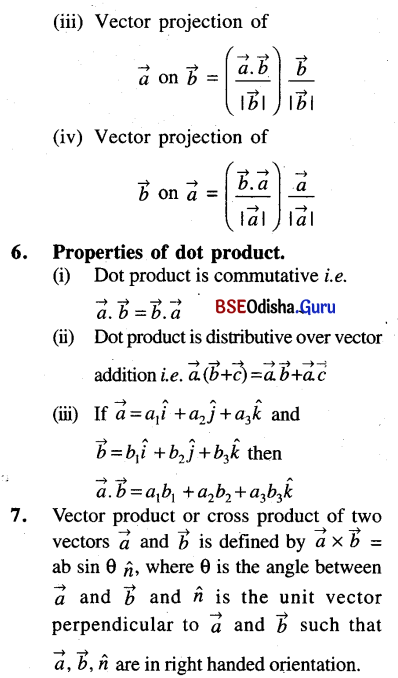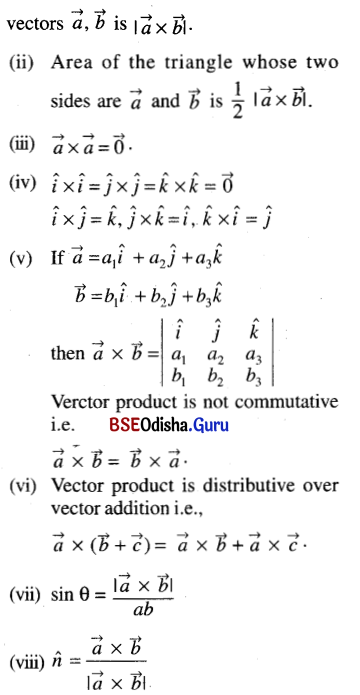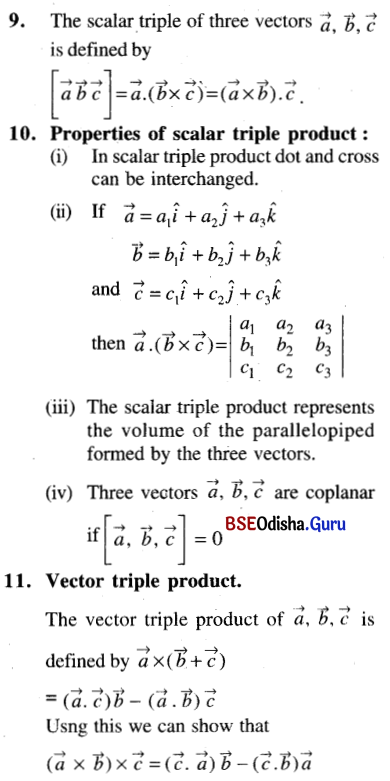# CHSE Odisha Class 12 Math Notes Chapter 12 Vectors

Odisha State Board CHSE Odisha Class 12 Math Notes Chapter 12 Vectors will enable students to study smartly.

## CHSE Odisha 12th Class Math Notes Chapter 12 Vectors

Important formulae:
1. If P = (x1, y1, z1) and Q = (x2, y2, z2) then
P͞Q = (x2 – x1) î + (y2 – y1 ) ĵ + (z2 – z1) k̂
where î, ĵ, k̂ are the unit vectors along x-axis, y-axis and z-axis.

2. Magnitude of a vector:8. Properties of vector product:
(i) Area of a parallelogram whose adjacent sides are represented by the12. The vector equation of a straight line:
(i) The vector equation of a straight line passing through a point with position vector $$\vec{a}$$ and parallel to a vector $$\vec{b}$$ is $$\vec{r}=\vec{a}+t \vec{b}$$ where t is a parameter.
(ii) The equation ofa striaght line through two points with position vectors $$\vec{a}$$ and $$\vec{b}$$ is $$\vec{r}=\vec{a}+t(\vec{b}-\vec{a})$$.
(iii) Equation of a straight line through a point with position vector $$\vec{a}$$ and perpendicualr to two non-parallel $$\vec{b}$$ and $$\vec{c}$$ is $$\vec{r}=\vec{a}+t(\vec{b} \times \vec{a})$$.13. The vector equation of a plane:
(i) The vector equation of plane through a point $$\vec{a}$$ and perpendicular to n̂ is $$(\vec{r}-\vec{a}) \cdot \hat{n}$$ = 0
(ii) The equation of a plane through a point $$\vec{a}$$ and parallel to non-parallel vectors $$\vec{b}$$ and $$\vec{c}$$ is $$\vec{r}=\vec{a}+t \vec{b}+s \vec{c}$$, where t and s are parameters.
(iii) Equation of the plane passing through the points $$\vec{a}, \vec{b}$$ and parallel to $$\vec{c}$$ is $$\vec{r}=(1-t) \vec{a}+t \vec{b}+s \vec{c}$$.
(iv) Equation of the plane through three non collinear points $$\vec{a}, \vec{b}, \vec{c}$$ is $$\vec{r}=(1-s-t) \vec{a}+t \vec{b}+s \vec{c}$$.